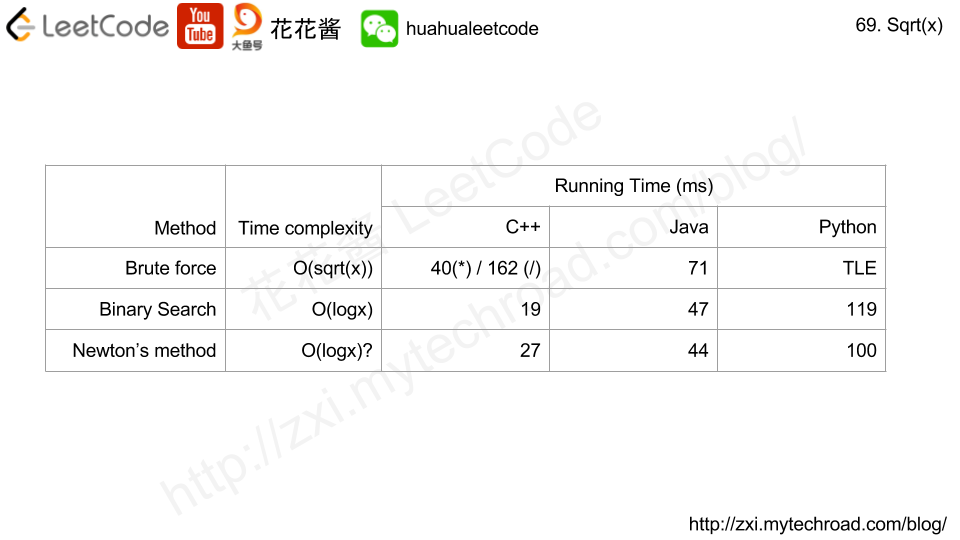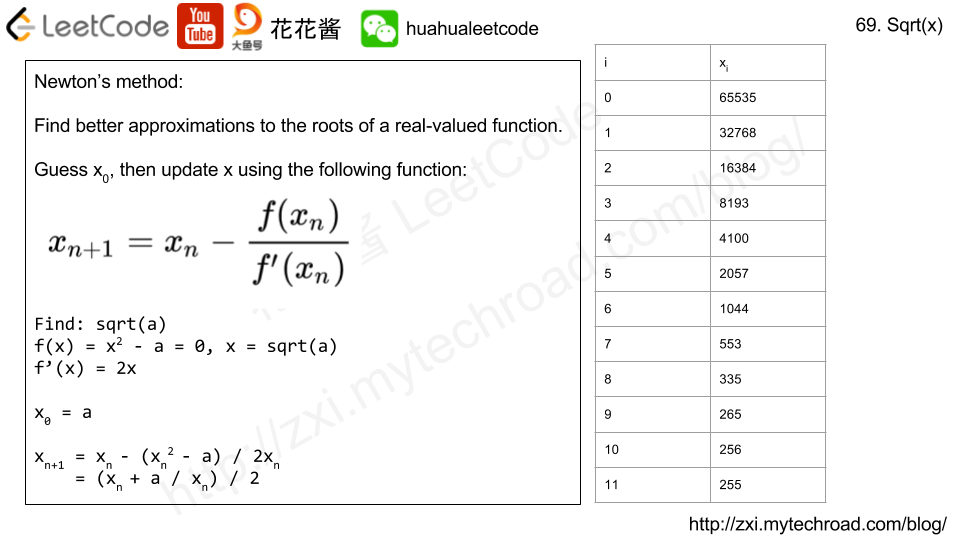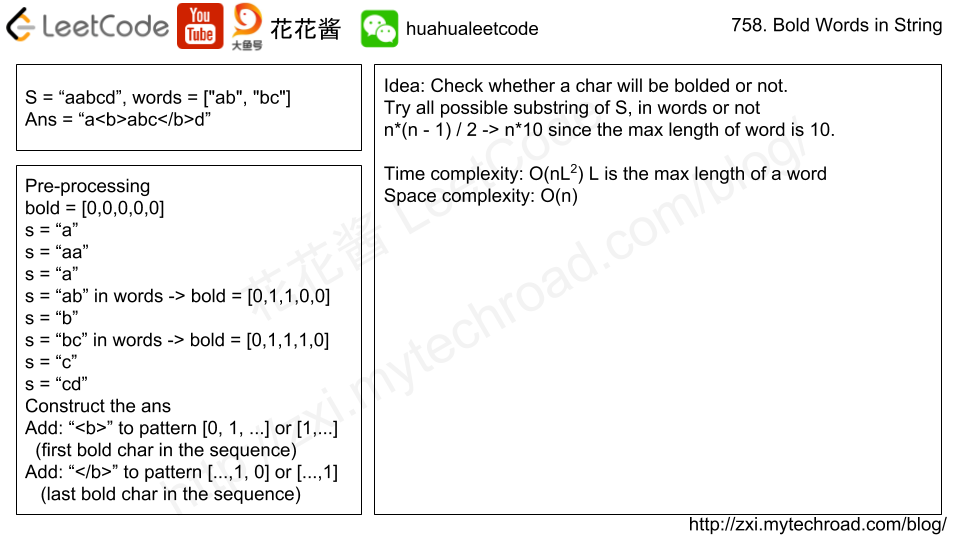# Posts published in “Easy”

Given a Binary Search Tree (BST) with the root node root, return the minimum difference between the values of any two different nodes in the tree.

Example :

Note:

1. The size of the BST will be between 2 and 100.
2. The BST is always valid, each node’s value is an integer, and each node’s value is different.

Solution 1: In order traversal

Time complexity: O(n)

Space complexity: O(n)

C++

Related Problems:

A matrix is Toeplitz if every diagonal from top-left to bottom-right has the same element.

Now given an M x N matrix, return True if and only if the matrix is Toeplitz.
Example 1:

Example 2:

Note:

1. matrix will be a 2D array of integers.
2. matrix will have a number of rows and columns in range [1, 20].
3. matrix[i][j] will be integers in range [0, 99].

Idea:

Check m[i][j] with m[i-1][j-1]

Solution: Brute Force

Time complexity: O(n*m)

Space complexity: O(1)

C++

Java

Python3

Given two integers L and R, find the count of numbers in the range [L, R] (inclusive) having a prime number of set bits in their binary representation.

(Recall that the number of set bits an integer has is the number of 1s present when written in binary. For example, 21 written in binary is 10101 which has 3 set bits. Also, 1 is not a prime.)

Example 1:

Example 2:

Note:

1. L, R will be integers L <= R in the range [1, 10^6].
2. R - L will be at most 10000.

Solution 1: Brute Force

C++

Java

Python2

Python2

Problem:

Implement int sqrt(int x).

Compute and return the square root of x.

x is guaranteed to be a non-negative integer.

Example 1:

Example 2:# Solution 1: Brute force

Time complexity: sqrt(x)

# Solution 2: Binary search

Time complexity: O(logn)

# Solution 3: Newton’s method

## Python3

Problem:

Given a set of keywords words and a string S, make all appearances of all keywords in S bold. Any letters between <b> and </b> tags become bold.

The returned string should use the least number of tags possible, and of course the tags should form a valid combination.

For example, given that words = ["ab", "bc"] and S = "aabcd", we should return "a<b>abc</b>d". Note that returning "a<b>a<b>b</b>c</b>d" would use more tags, so it is incorrect.

Note:

1. words has length in range [0, 50].
2. words[i] has length in range [1, 10].
3. S has length in range [0, 500].
4. All characters in words[i] and S are lowercase letters.Solution:

C++

Time complexity: O(nL^2)

Space complexity: O(n + d)

d: size of dictionary

L: max length of the word which is 10.

Mission News Theme by Compete Themes.